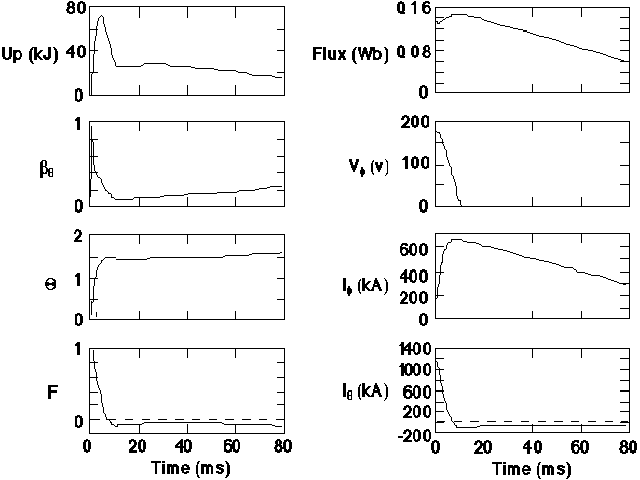# Modified Polynomial Function Model for Reversed-field Pinches

W. Shen and J. C. Sprott
Department of Physics, University of Wisconsin, Madison, Wisconsin 53706
(Received 26 Nobember 1990; accepted 29 January 1991)

### ABSTRACT

A generalized analytic representation for the magnetic field and current density profiles in a reversed-field pinch (RFP) is proposed. These profiles have zero current density at the wall and finite plasma pressure. The profiles are characterized by two free parameters here taken to be the field-reversal parameter (F) and pinch parameter (theta). From the profiles, many useful quantities such as magnetic energy, beta, inductance, resistance, and Ohmic input power are calculated. These quantities provide a basis for analyzing experimental data and performing electrical circuit modeling of RFP discharges.

Ref: W. Shen and J. C. Sprott, Phys. Fluids B 3, 1225-1231 (1991)

The complete paper is available in PDF format.

Fig. 1. Contours of constant asymmetry factor in F-Theta space as predicted by the modified polynomial function model.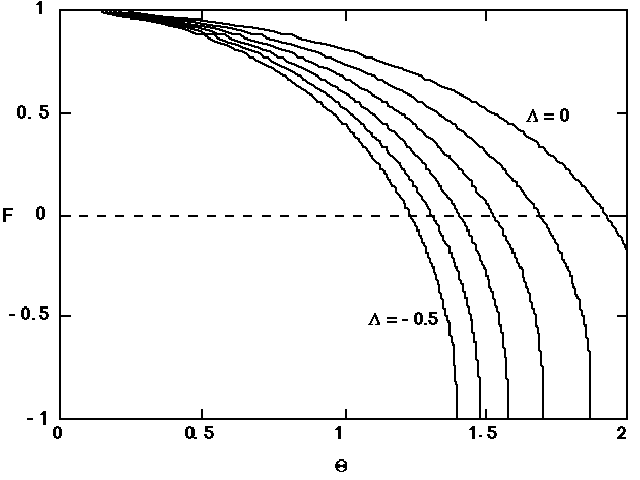Fig. 2. Magnetic field profiles measured in MST (crosses) compared with the predictions of the modified polynomial function model (solid curves).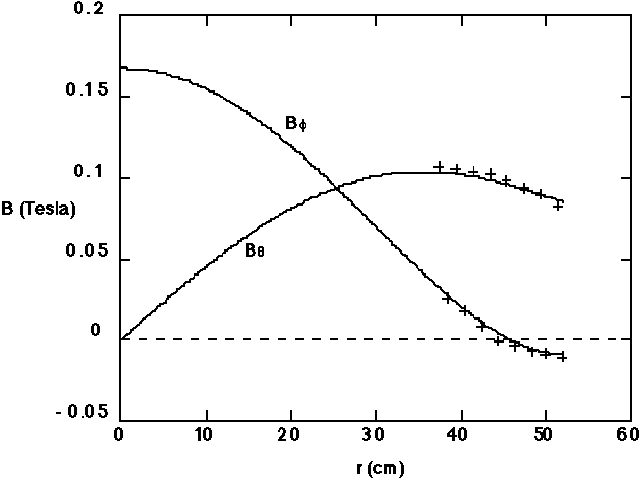Fig. 3. The F-Theta plot for the modified polynomial function model at various values of beta-theta compared with the Bessel function model (BFM) and the polynomial function model (PFM).Fig. 4. Normalized contours of magnetic energy in F-Theta space as predicted by the modified polynomial function model.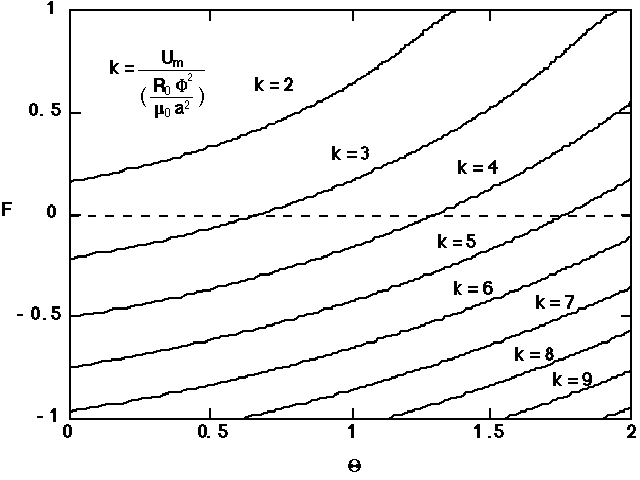Fig. 5. Normalized self-inductance of an RFP plasma with fixed I-theta and Phi.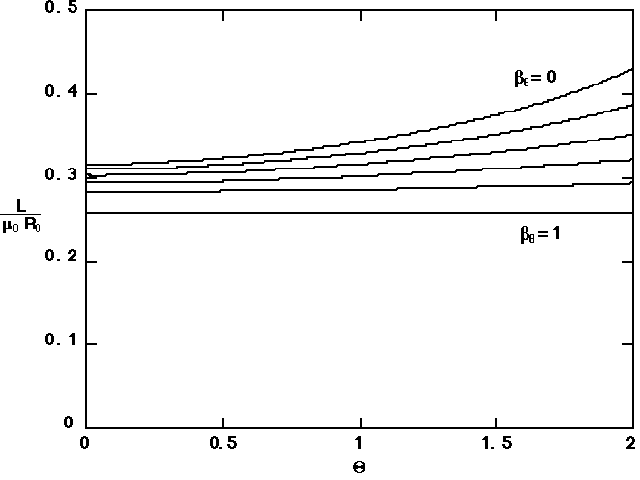Fig. 6. Normalized mutual inductance of an RFP plasma with fixed I-theta and Phi.Fig. 7. Normalized coupling coefficient of an RFP plasma with fixed I-theta and I-phi.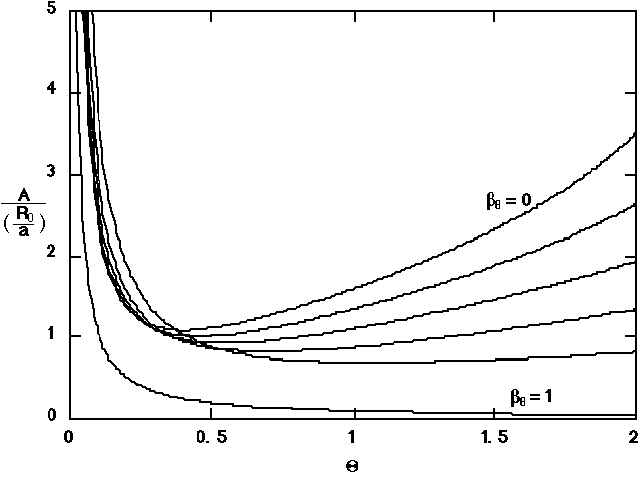Fig. 8. Normalized resistance of an RFP plasma with a spatially independent parallel resistivity.Fig. 9. Numerical solutions of the time-dependent MPFM circuit equations for a case resembling the MST experiment.